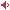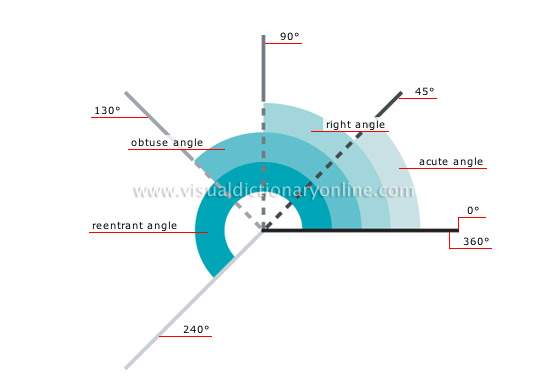Visual Dictionary Online

Theme

examples of anglesAngle: figure formed by two intersecting lines or planes; it is measured in degrees.reentrant angleAngle between 180° and 360°.

obtuse angleAngle between 90° and 180°.

right angleAngle formed by two lines or two perpendicular planes that measures 90°.

acute angleAngle that is smaller than a right angle (less than 90°).